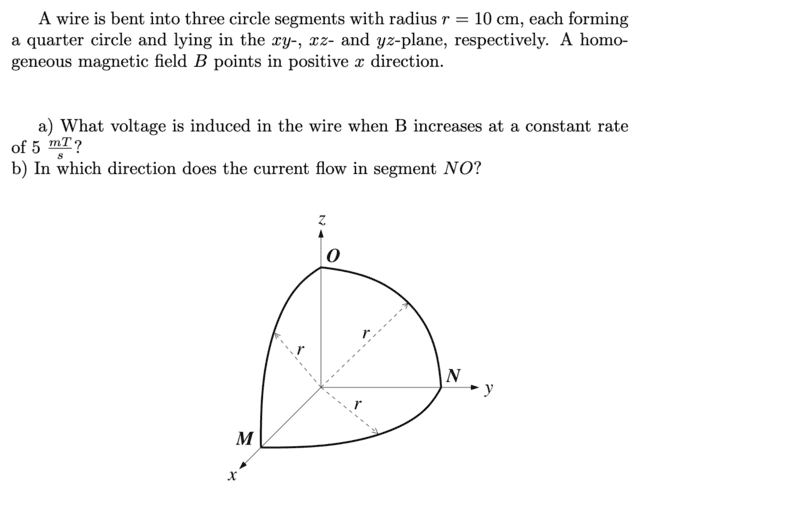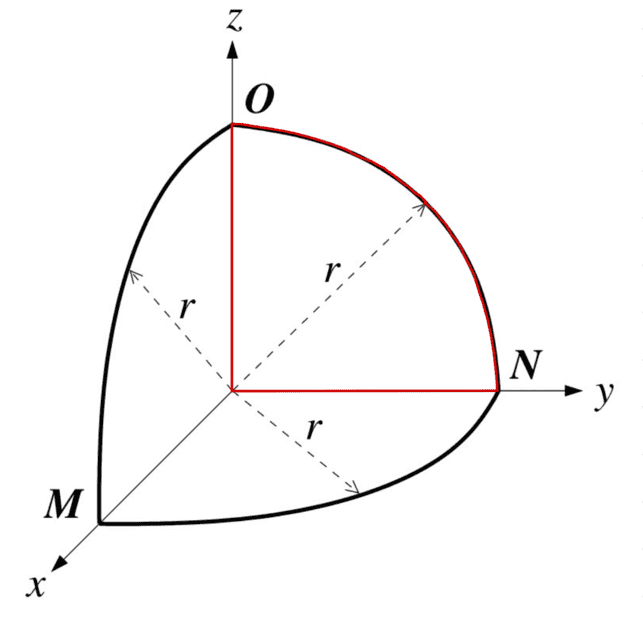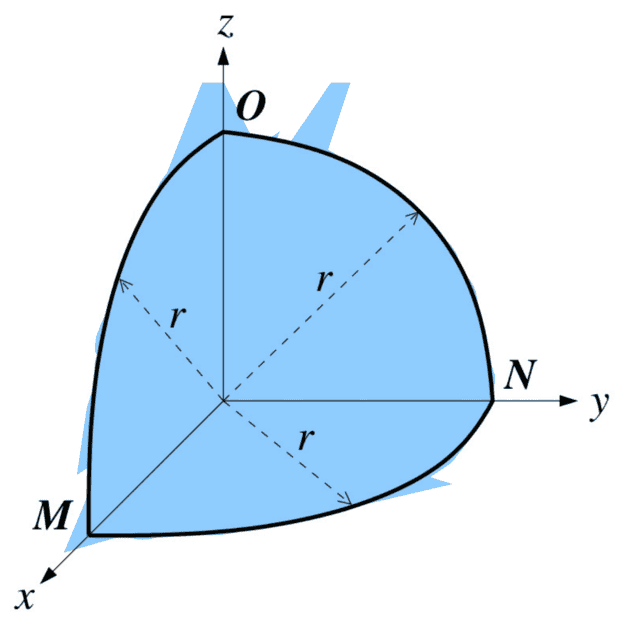# Induction in a bent wire

• Lambda96
In summary, the magnetic flux does flow through the blue surface corresponding to the surface of a ##\frac{1}{8}## sphere. The induced voltage is calculated to be 3.92*10^{-5} V.f

#### Lambda96

Homework Statement
What voltage is induced in the bent wire
Relevant Equations
none
Hi,

unfortunately, I am not sure if I have calculated the task correctly hereI have now proceeded in such a way that I thought that the magnetic field only flows through the area drawn in red. Which ##\frac{1}{4}## corresponds to the area of a circle.By the fact that the magnetic field is constant and points in direction x, that the magnetic field is parallel to the normal vector of the surface. Then the following applies ##\vec{B} \cdot d \vec{A}=B \cdot dA##.

Then the magnetic flux is as follows

$$\phi =\int_{}^{} B dA=\frac{1}{4} \pi r^2 B$$

The induced voltage is then calculated as

$$\frac{d \phi}{dt}=\frac{1}{4} \pi r^2 \frac{dB}{dt}=\frac{1}{4} \pi (0.1m)^2 5 \frac{mT}{s}=3.92*10^{-5} V$$

The induced current always flows in such a way that an opposing magnetic field is generated to neutralize the changing external magnetic field, which means that the current in the NO segment must flow clockwise.

I am not sure if I have solved the problem a correctly, because does the magnetic flux also flow, through the blue colored surface, which corresponds to the surface of a ##\frac{1}{8}## a sphere.The magnetic field is constant in x-direction, that means the normal vector of the surface and the magnetic field is not parallel to each other and therefore the calculation would be much more complicated.

In calculating the induced emf, you must choose a surface for the flux that is bounded by the three circular arcs. For example, the 1/8 of the spherical surface that you colored in blue would be acceptable except that it is not convenient (as you noticed).

You chose a surface that is bounded by the y-axis, the z-axis, and the arc ON. So, this surface by itself does not qualify. Can you see how to "add some more surface" to your choice so that you get a surface that is bounded by all three arcs and allows you to see that your answer for the emf is still correct?

•Lambda96
I'm getting the same thing as you for Part A and I carried out the calculation in spherical coordinates. This is how you would do the calculation long hand if you wanted to.

##\epsilon = - \frac{d \Phi_B}{dt}##

##\vec{B} = \left( 5 \cdot 10^{-3} t, 0 , 0 \right)## in Cartesian

##\Phi_B = \oint \vec{B} \cdot d\vec{a}##

Looking in the back cover of Griffiths ##\hat{x}## in Spherical basis is the following

##\hat{x} = \sin \theta \cos \phi \hat{r} + \cos \theta \cos \phi \hat{\theta} - \sin \phi \hat{\phi}##

So ##\vec{B}## in spherical basis is

##\vec{B} = \left( 5 \cdot 10^{-3} t\right)\left[ \sin \theta \cos \phi \hat{r} + \cos \theta \cos \phi \hat{\theta} - \sin \phi \hat{\phi}\right]##

We know that the differential volume element for a sphere is

##dV = r^2 \sin \theta dr d\theta d\phi##

##da## is the same as ##dV## with ##dr## taken away

##da = r^2 \sin \theta d\theta d\phi##

##d\vec{a}## is in the ##\hat{r}## direction so ##d\vec{a} = r^2 \sin \theta d\theta d\phi \hat{r}##

so

## \vec{B} \dot d\vec{a} = \left( 5 \cdot 10^{-3} t \right) r^2 \sin^2 \theta \cos \phi d\theta d\phi##

##\Phi_B = \left( 5 \cdot 10^{-3} \right) r^2 \int_{\phi = 0}^{\frac{pi}{2}} \int_{\theta= 0}^{\frac{pi}{2}} \sin^2 \theta \cos \phi \, d\theta d \phi##

## = \left( 5 \cdot 10^{-3} t \right) \left(0.10\right)^2 \left( \int_{0}^{\frac{pi}{2}} \sin^2 \theta \,d\theta\right) \left( \int_{0}^{\frac{pi}{2}} \cos \phi \,d \phi \right)##

Using a power reduction formula on the ##\sin^2 \theta## and cranking out the rest of the integral(s)

##\Phi_B = \left( 5 \cdot 10^{-3} t \right) \left(0.10\right)^2 \left(\frac{pi}{4}\right)##

##-\frac{\Phi_B}{dt} = \left( 5 \cdot 10^{-3} \right) \left(0.10\right)^2 \left(\frac{pi}{4}\right) = 3.92 \cdot 10^{-5} V##

•Lambda96
It can be any surface enclosed by the wires. Your original argument is correct.
For the 3 separate surfaces on the planes.
Let A=(##π/4##)r2
##\vec A## 1=A##\hat i##
##\vec A## 2=A##\hat j##
##\vec A## 3=A##\hat k##
And,
##\vec B##=(5x10-3t)##\hat i## (t=time)

##\vec B## ##\cdot## ##\vec A## 1=(5x10-3t)(##π/4##)r2
##\vec B## ##\cdot## ##\vec A## 2=0
##\vec B## ##\cdot## ##\vec A## 3=0

So,
##\Phi##=Σ##\vec B## ##\cdot## ##\vec A## i= (5x10-3t)(##π/4##)r2
which is the correct answer for the flux ##\Phi##.

If you use the spherical surface, there is no need to include the planar surface.
If you use the planar surface, there is no need to include the spherical surface.

Any single surface bounded by the wires will give the correct answer.

Since B is increasing in the x direction, a current from O to N would produce a magnetic field pointing in the negative x direction, opposing the change in flux.

•Lambda96 and PhDeezNutz
The induced current always flows in such a way that an opposing magnetic field is generated to neutralize the changing external magnetic field, ##\dots##
That is an incorrect and misleading statement. The induced current flows in a such a way as to oppose any proposed change in the external magnetic field. Specifically, if the external magnetic field is decreasing, the induced current will flow in a way that will reinforce, not "neutralize" the external field. You must be absolutely clear about this point.

•hutchphd and Lambda96
Thank you TSny, PhDeezNutz, harveysnaf and kuruman for your help, now I got itAlso thanks PhDeezNutz for your detailed calculation for the flux over the spherical surfaceAlso thanks kuruman for your hint, then I had misunderstood the induced current, now no more thanks to your help•TSny, PhDeezNutz and kuruman
I think it tacitly implied in these answers but should be stated that these considerations are all driven by the Maxwell equation prohibition$$\iint_{closed~S} \vec B \cdot d \vec S =0$$ So any closed surface can be usefully cut into pieces

•PhDeezNutz
I think it tacitly implied in these answers but should be stated that these considerations are all driven by the Maxwell equation prohibition$$\iint_{closed~S} \vec B \cdot d \vec S =0$$ So any closed surface can be usefully cut into pieces
I think this is really insightful. And it had slipped my mind. ##\nabla \cdot \vec{B} = 0## so by the divergence theorem as the flux through the surface (spherical surface in the first octant PLUS the coordinate plane intersections it is bounded by) is zero; Whatever comes in is whatever comes out so it is completely appropriate just to consider the flux through the quarter circle in the yz-plane considering the vector field is in the ##\hat{x}## direction.

So basically OP was right for the reasons you stated.

•hutchphd